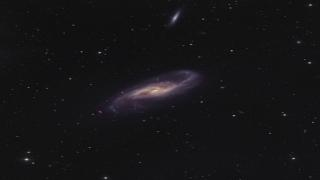# Fractal dimension of optical cirrus in Stripe82

Marchuk, Alexander A.; Smirnov, Anton A.; Mosenkov, Aleksandr V.; Il'in, Vladimir B.; Gontcharov, George A.; Savchenko, Sergey S.; Román, Javier
Referencia bibliográfica

Monthly Notices of the Royal Astronomical Society

Fecha de publicación:
12
2021
Descripción
The geometric characteristics of dust clouds provide important information on the physical processes that structure such clouds. One of such characteristics is the 2D fractal dimension D of a cloud projected on to the sky plane. In previous studies, which were mostly based on infrared (IR) data, the fractal dimension of individual clouds was found to be in a range from 1.1 to 1.7 with a preferred value of 1.2-1.4. In this work, we use data from Stripe82 of the Sloan Digital Sky Survey to measure the fractal dimension of the cirrus clouds. This is done here for the first time for optical data with significantly better resolution as compared to IR data. To determine the fractal dimension, the perimeter-area method is employed. We also consider IR (IRAS and Herschel) counterparts of the corresponding optical fields to compare the results between the optical and IR. We find that the averaged fractal dimension across all clouds in the optical is $\langle D \rangle =1.69^{+0.05}_{-0.05}$ which is significantly larger than the fractal dimension of its IR counterparts $\langle D\rangle =1.38^{+0.07}_{-0.06}$. We examine several reasons for this discrepancy (choice of masking and minimal contour level, image and angular resolution, etc.) and find that for approximately half of our fields the different angular resolution (point spread function) of the optical and IR data can explain the difference between the corresponding fractal dimensions. For the other half of the fields, the fractal dimensions of the IR and visual data remain inconsistent, which can be associated with physical properties of the clouds, but further physical simulations are required to prove it.